OliNo

Renewable Energy

Oxxy Light Led Tube 120cm Master Food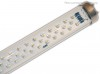presents a 120 cm long led tube with the name Oxxy Light. There are several variants of this tube, the warm white, the neutral white and the master food (specially for the meat industry). In this article the master food tube.

This article shows the measurement results. Many parameters are also found in the Eulumdat file.

See this overview for a comparison with other light bulbs.

Summary measurement data

parameter meas. result remark
Color temperature 3882 K Is not white but has a reddish color
Luminous intensity Iv 426 Cd Measured straight underneath the lamp
Beam angle 119 deg 119º for the C0-C180 plane (crosses the length direction of the tube), and 115º for C90-C270 plane (along the length direction of tube).
Power P 13.8 W
Power Factor 0.78 For every 1 kWh net power consumed, there has been 0.8 kVAhr for reactive power.
Luminous flux 1413 Lm
Luminous efficacy 102 Lm/W
CRI_Ra 71 Color Rendering Index.
Coordinates chromaticity diagram x=0.3701 and y=0.3225
Fitting FL-tube This tube is directly connected to the mains voltage (230V).
PAR-value 4.1 μMol/s/m2 The number of photons seen by an average plant when it is lit by the light of this light bulb. Value valid at 1 m distance from light bulb.
S/P ratio 1.5 This factor indicates the amount of times more efficient the light of this light bulb is perceived under scotopic circumstances (ow environmental light level).
D x L external dimensions 30 x 1200 mm External dimensions of the tube (D = diameter). Excluding the pins.
L x W luminous area 1155 x 20 mm Dimensions of the luminous area (used in Eulumdat file). This is equal to the surface of the plate on which the leds are mounted.
General remarks The ambient temperature during the whole set of measurements was 23.5-25.5 deg C.

Warm up effect: during the warm up time the illuminance decreases with ≈11 % and the consumed power with ≈12 %.

Voltage dependency: the power consumption and illuminance do depend on the voltage when it is varied from 200 – 250 V.

Measurement report (PDF)Eulumdat fileRight click on icon and save the file.

Overview table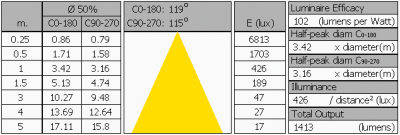The overview table is explained on the OliNo website. Please note that this overview table makes use of calculations, use this data with care as explained on the OliNo site. E (lux) values are not accurate, as the distances 0.25 – 5 m are in the near field of the tube; real measured lux values will be lower than here computed.

Eulumdat light diagram

This light diagram below comes from the program Qlumedit, that extracts these diagrams from an Eulumdat file. It is explained on the OliNo site.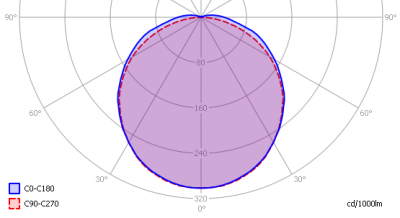The light diagram giving the radiation pattern.

It indicates the luminous intensity around the light bulb. In the length direction (C90-C270) the beam angle is almost the same as in the direction crossing the length direction (C0-C180).

Illuminance Ev at 1 m distance, or luminous intensity Iv

Herewith the plot of the averaged luminous intensity Iv as a function of the inclination angle with the light bulb.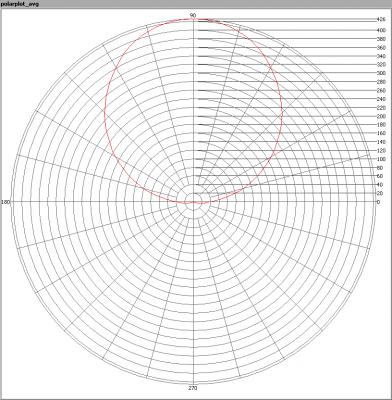The radiation pattern of the light bulb.

This radiation pattern is the average of the light output of the light diagram given earlier. Also, in this graph the luminous intensity is given in Cd.

These averaged values are used (later) to compute the lumen output.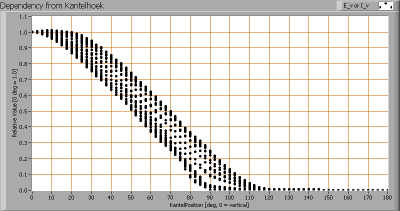Intensity data of every measured turn angle at each inclination angle.

This plot shows per inclination angle the intensity measurement results for each turn angle at that inclination angle. There normally are differences in illuminance values for different turn angles. However for further calculations the averaged values will be used.

When using the average values per inclination angle, the beam angle can be computed, being 115-119º depending on the reference plane.

Luminous flux

With the averaged illuminance data at 1 m distance, taken from the graph showing the averaged radiation pattern, it is possible to compute the luminous flux.

The result of this computation for this light spot is a luminous flux of 1413 Lm.

Luminous efficacy

The luminous flux being 1413 Lm, and the power of the light bulb being 13.8 W, yields a luminous efficacy of 102 Lm/W.

A power factor of 0.78 means that for every 1 kWh net power consumed, a reactive component of 0.8 kVAr was needed.

 Light bulb voltage (used on power supply!) 230 VAC Light bulb current 77 mA Power P 13.8 W Apparent power S 17.8 VA Power factor 0.78

Of this light bulb the voltage across ad the resulting current through it are measured and graphed. See the OliNo site how this is obtained.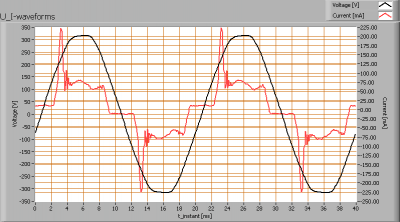Voltage across and current through the lightbulb

The current does not have a sine form, but tries to approach it. The phase of the current is a bit leading the phase of the voltage.The powerfactor is good, about 0.8.

Also the power spectrum of the current is determined.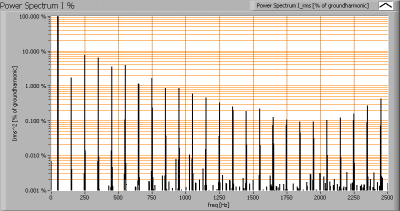Squared amplitudes of higher harmonics related to the first harmonic (50 Hz).

Due to steep edges in the current, there are manu higher harmonics.

The Total Harmonic Distortion of hte current is 60 %.

Color temperature and Spectral power distribution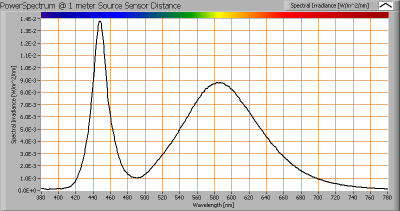The spectral power distribution of this light bulb, energies on y-axis valid at 1 m distance.

The measured color temperature is about 3875 K which is neutral white.

This color temperature is measured straight underneath the light bulb. Below a graph showing the color temperature for different inclination angles.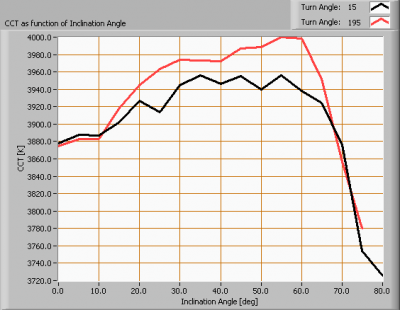Color temperature as a function of inclination angle.

The measurement of CCT is measured for inclination angles up to 75º since then the illuminance value was decreased to very low values (< 5 lux).

The beam angle is maximally 119º, meaning a 59.5º inclination angle. In this area the majority of the light is present. The variation in correlated color temperature in this area is about 3 %.

PAR value and PAR spectrum

To make a statement how well the light of this light bulb is for growing plants, the PAR-area needs to be determined. See the OliNo website how this all is determined and the explanation of the graph.The photon spectrum, then the sensitivity curve and as result the final PAR spectrum of the light of this light bulb

The PAR number for this light results in 4.1 μMol/s/m2. This value is valid at 1 m distance from the light bulb and valid roughly inside its beam angle.

The PAR efficiency is 66 % (valid for the PAR wave length range of 400 – 700 nm). So maximally 66 % of the total of photons in the light is effectively used by the average plant (since the plant might not take 100 % of the photons at the frequency where its relative sensitivity is 100 %).

S/P ratio

The S/P ratio and measurement is explained on the OliNo website. Here the results are given.The power spectrum, sensitivity curves and resulting scotopic and photopic spectra (spectra energy content defined at 1 m distance).

The S/P ratio is 1.5

Chromaticity diagram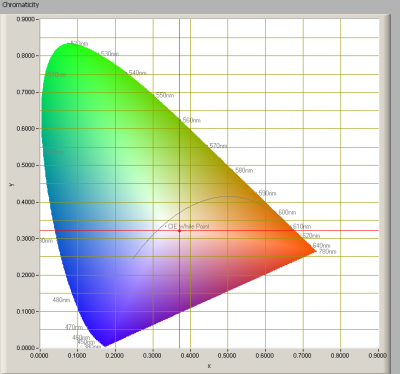The chromaticity space and the position of the lamp’s color coordinates in it.

The light coming from this lamp is far away from the Planckian Locus (the black path in the graph). It is understandable, as this tube has a light comptimized for illuminating red meat, hence the color of this light is not white but more reddish.

Its coordinates are x=0.3701 and y=0.3225.

Color Rendering Index (CRI) or also Ra

Herewith the image showing the CRI as well as how well different colors are represented (rendered). The higher the number, the better the resemblance with the color when a black body radiator would have been used (the sun, or an incandescent lamp). Practical information and also some critics about the CRI can be found on the OliNo website.

Each color has an index Rx, and the first 8 indexes (R1 .. R8) are averaged to compute the Ra which is equivalent to the CRI.CRI of the light of this lightbulb.

The value of 71 is lower than 80 which is considered a minimum value for indoor usage.

Note: the chromaticity difference is 0.025 indicates the distance to the Planckian Locus. Its value is higher than 0.0054, which means that the calculated CRI result is not meaningful. The color of the light cannot be considered as white. Which is correct, as this tube is used for illuminating well red meats, hence the color is reddish.

Voltage dependency

The dependency of a number of lamp parameters on the lamp voltage is determined. For this, the lamp voltage has been varied and its effect on the following light bulb parameters measured: illuminance E_v [lx], the lamppower P [W], the (Correlated) Color Temperature [K] and the luminous efficacy [Lm/W].Lamp voltage dependencies of certain light bulb parameters, where the value at 230 V is taken as 100 %.

The illuminance and consumed power do vary linearly when the voltage is varied.

When the voltage at 230 V varies with + and – 5 V, then the illuminance varies ≈ 2 %, so when abrupt voltage changes occur this effect is not visible in the illuminance output.

Warm up effects

After switch on of a cold lamp, the effect of heating up of the lamp is measured on illuminance E_v [lx], color temperature CT or correlated color temperature CCT [K], the lamppower P [W] and the luminous efficacy [lm/W].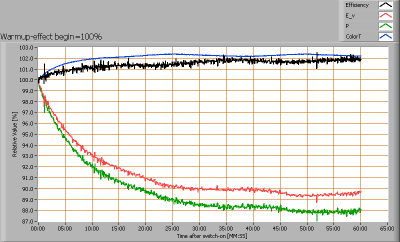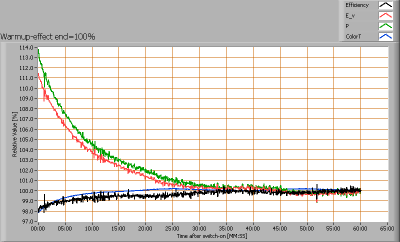Effect of warming up on different light bulb parameters. At top the 100 % level is put at begin, and at bottom at the end.

The warm up time is about 30 minutes. During that time the illuminance decreases with ≈ 11 % and the consumed power with ≈ 12 %.